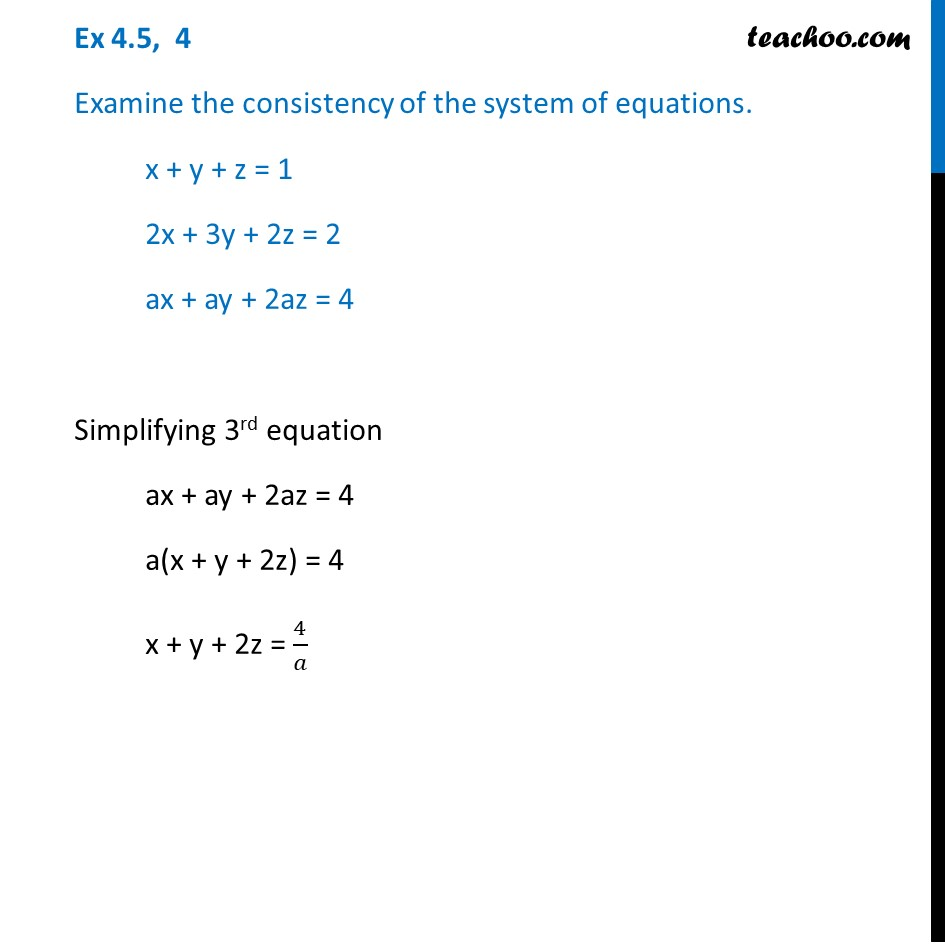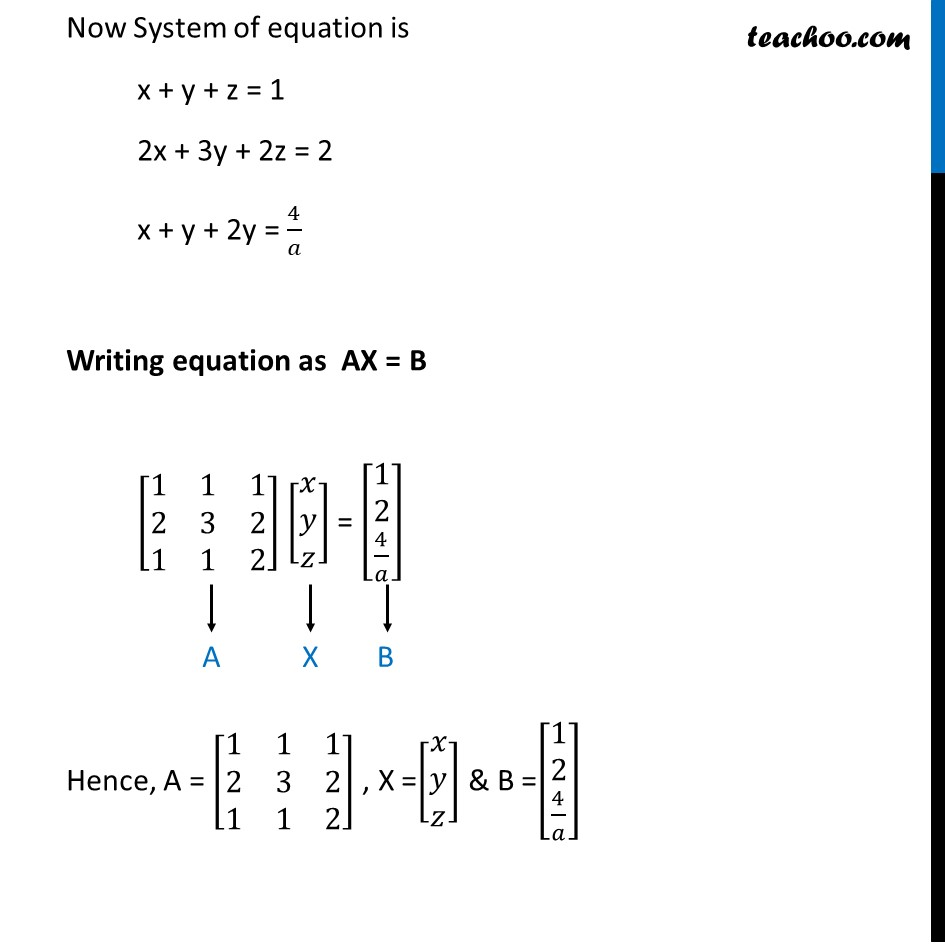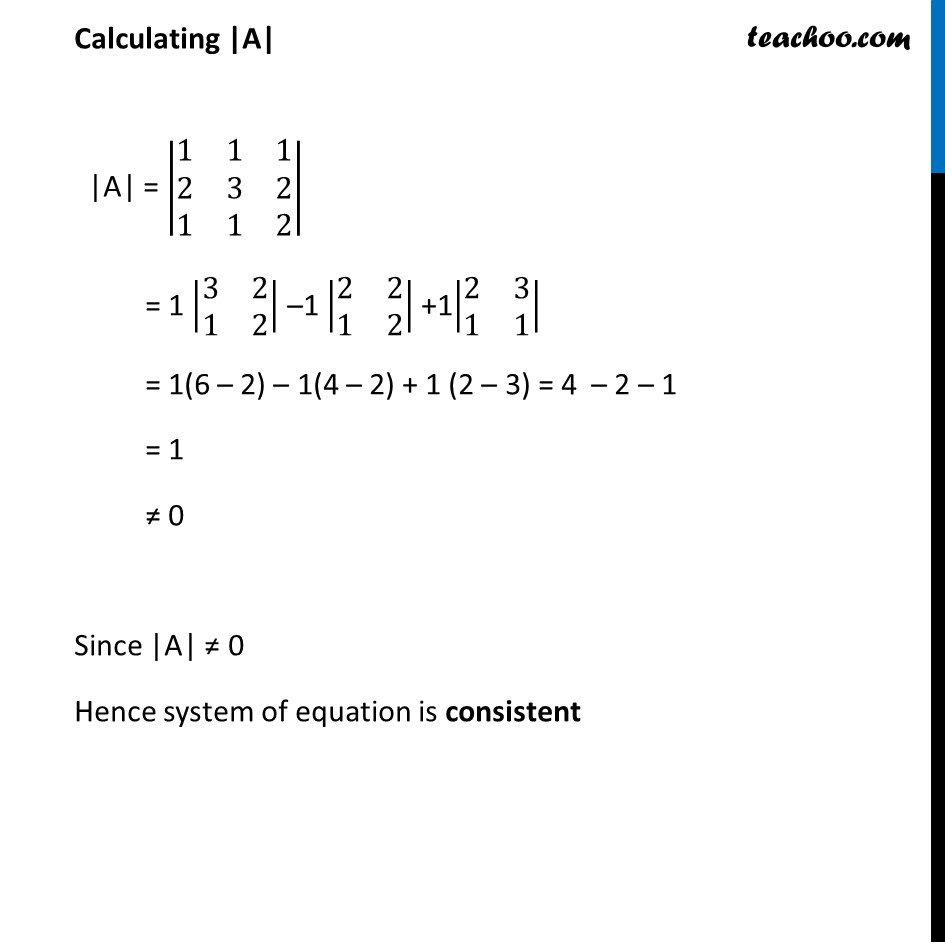Ex 4.5

Chapter 4 Class 12 Determinants
Serial order wiseLearn in your speed, with individual attention - Teachoo Maths 1-on-1 Class

### Transcript

Ex 4.5, 4 Examine the consistency of the system of equations. x + y + z = 1 2x + 3y + 2z = 2 ax + ay + 2az = 4 Simplifying 3rd equation ax + ay + 2az = 4 a(x + y + 2z) = 4 x + y + 2z = 4/𝑎 Now System of equation is x + y + z = 1 2x + 3y + 2z = 2 x + y + 2y = 4/𝑎 Writing equation as AX = B [■8(1&1&1@2&3&2@1&1&2)] [■8(𝑥@𝑦@𝑧)] = [■8(1@2@4/𝑎)] Hence, A = [■8(1&1&1@2&3&2@1&1&2)] , X =[■8(𝑥@𝑦@𝑧)] & B =[■8(1@2@4/𝑎)] Calculating |A| |A| = |■8(1&1&1@2&3&2@1&1&2)| = 1 |■8(3&2@1&2)| –1 |■8(2&2@1&2)| +1|■8(2&3@1&1)| = 1(6 – 2) – 1(4 – 2) + 1 (2 – 3) = 4 – 2 – 1 = 1 ≠ 0 Since |A| ≠ 0 Hence system of equation is consistent